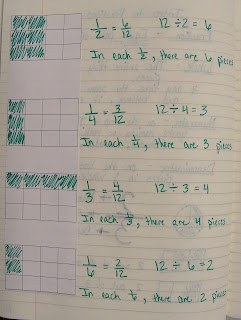## Friday, November 9, 2012

### Intro to FractionsFraction Basics

In math we have started to discuss fractions. By 4th grade, the kiddos have had a ton of practice naming fractions of a shape, as well as naming fractions of a set of objects. We continued that practice and discussed the definitions of the numerator and denominator. We've also extended our knowledge by figuring out how to find fractions of a number, such as 12 and 24.
We began by pulling out 12 squares and physically dividing them up into equal groups. It didn't take the kids long to figure out that to find fractions of a number, all they had to do was divide the number by the denominator of the fraction they were looking to find. For example, if they want to find 1/4 of 24, they could just divide 24 into 4 equal groups. Since 6 would be in each group, 1/4 of 24 is 6. Finally, we discussed how, if we found 1/4, we could also find 2/4, 3/4, etc.Finding fractions of 12 with blocksFinding fractions of 24Finding fractions of 24